Open in App
Not now

# Class 8 RD Sharma Solutions – Chapter 24 Data Handling II (Graphical Representation of Data as Histograms) – Exercise 24.1 | Set 1

• Last Updated : 19 Apr, 2021

### Draw a histogram representing the above data.

Solution:

To draw a histogram first construct x-axis and y-axis, where the x-axis represents class interval and the y-axis represents the frequency. Now, by taking suitable intervals from the given frequency table, we construct the rectangles as shown in the below figure.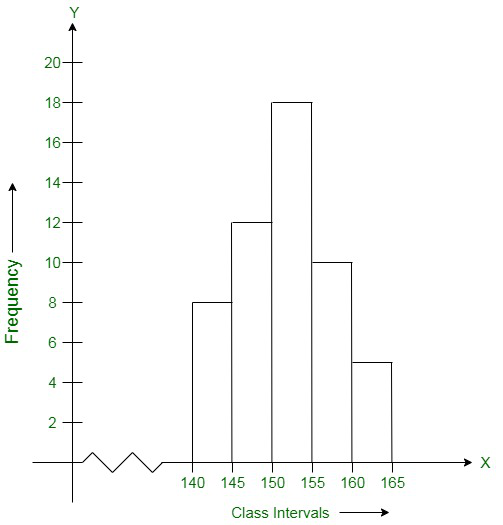### Question 2. Draw a histogram of the following data:

Solution:

To draw a histogram first construct x-axis and y-axis, where the x-axis represents class interval and the y-axis represents the frequency. Now, by taking suitable intervals from the given frequency table, we construct the rectangles as shown in the below figure.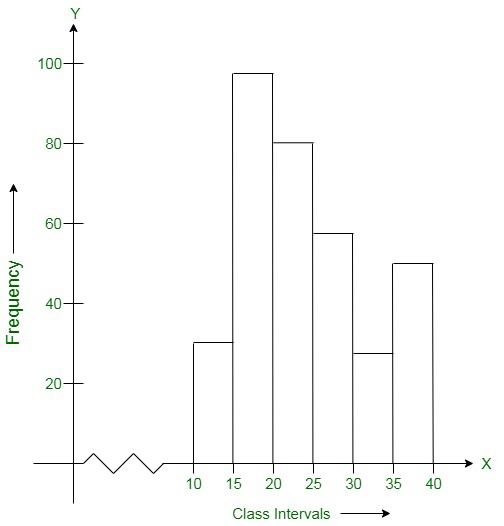### Draw a histogram representing the above data.

Solution:

To draw a histogram first construct x-axis and y-axis, where the x-axis represents class interval and the y-axis represents the frequency. Now, by taking suitable intervals from the given frequency table, we construct the rectangles as shown in the below figure.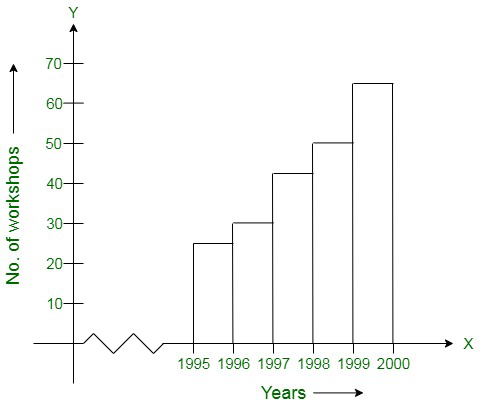### Draw the histogram of the frequency distribution (taking one of the class intervals as 50-100).

Solution:

First we create a frequency table using the given observations:

Now we draw a histogram using the frequency table:

To draw a histogram first construct x-axis and y-axis, where the x-axis represents class interval and the y-axis represents the frequency. Now, by taking suitable intervals from the given frequency table, we construct the rectangles as shown in the below figure.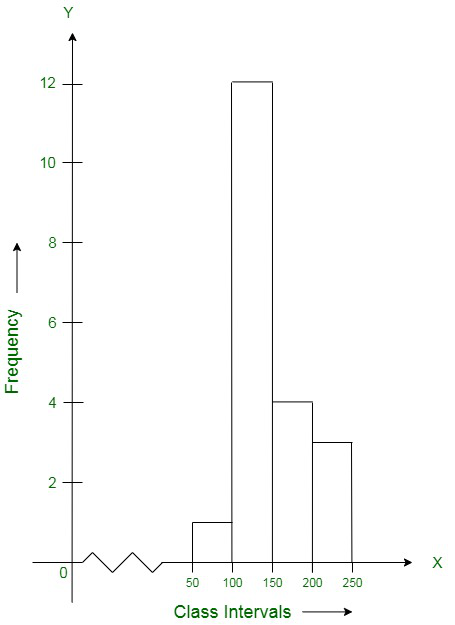### Question 5. Construct a histogram for the following data:

Solution:

To draw a histogram first construct x-axis and y-axis, where the x-axis represents monthly school fee(in Rs) and y-axis represents the no. of schools. Now, by taking suitable intervals from the given frequency table, we construct the rectangles as shown in the below figure.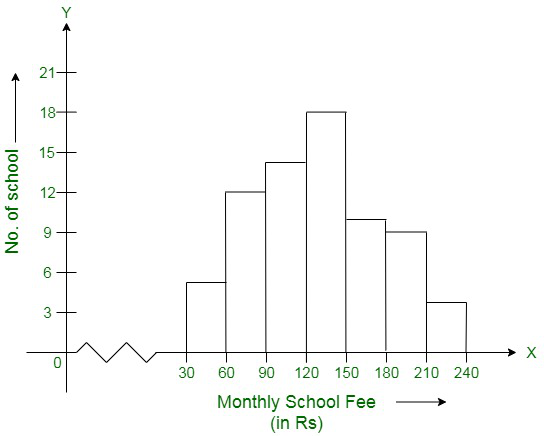### Question 6. Draw a histogram for the daily earnings of 30 drug stores in the following table:

Solution:

To draw a histogram first construct x-axis and y-axis, where x-axis represents monthly daily earnings(in Rs) and y-axis represents the no. of stores. Now, by taking suitable intervals from the given frequency table, we construct the rectangles as shown in the below figure.### Chapter 24 Data Handling II (Graphical Representation of Data as Histograms) – Exercise 24.1 | Set 2

My Personal Notes arrow_drop_up